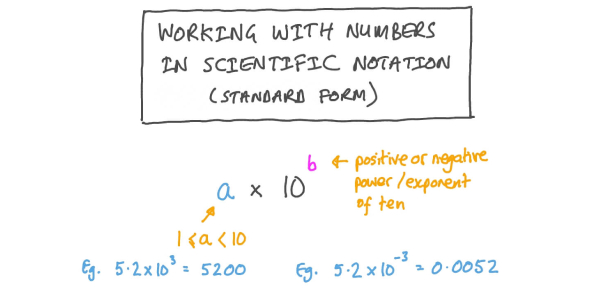# Trivia: Exponents, Scientific Notation, Cube Roots! Math Quiz

34 Questions | Attempts: 143
ShareSettingsHow much do you know about exponents, scientific notation, and cube roots? Exponents are numbers that you repeatedly multiply by the number in the exponent. Scientific notation is a way of expressing too large or too small numbers to be written in decimal form. A cube root is a value which when multiplied by itself three times, produces the original value. You absolutely must take this impeccable quiz.Back to top